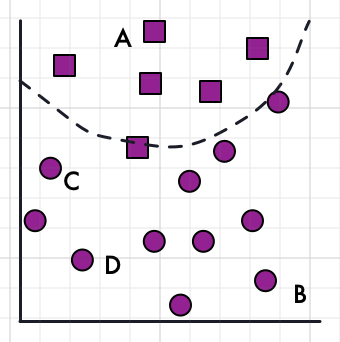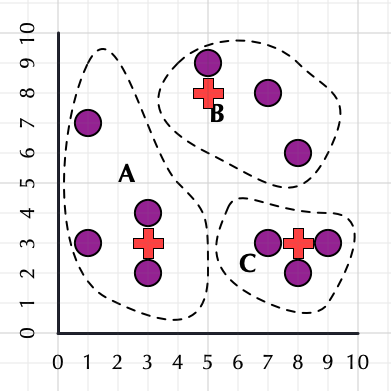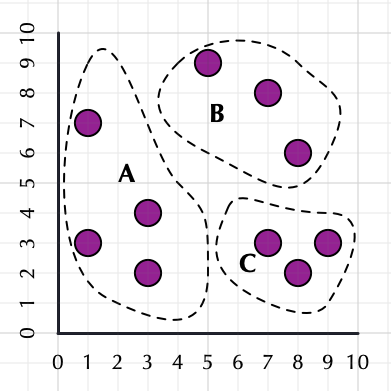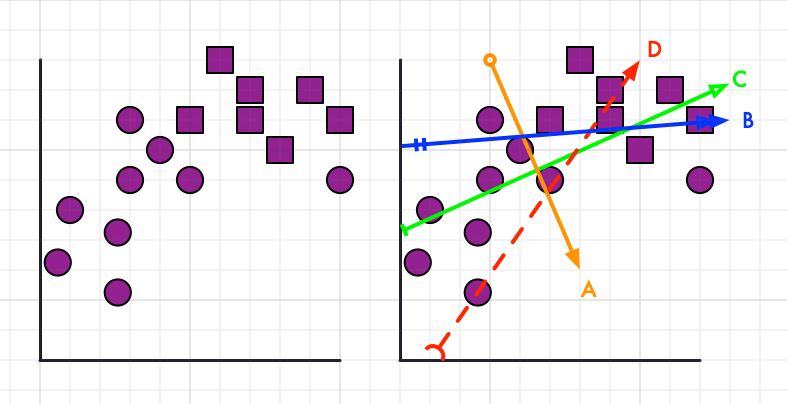Your browser doesn't support the features required by impress.js, so you are presented with a simplified version of this presentation.

For the best experience please use the latest Chrome, Safari or Firefox browser.

# ECE 657A Winter 2021 - Test 2

## Scope:

Content from live lectures and self-study videos from weeks 5 - 9 inclusive (but not including Deep Learning)

## Topics:

Streaming Ensemble Methods. Feature Extraction, Dimensionality Reduction, Word Embeddings, SVM, Clustering Algorithms and Evaluation Measures, Anomaly Detection

## Date and Time:

Was originally scheduled for March 11, will now be March 18-20

• you will have a 72 period to start the test, March 18-20
• then you will have a 3-hour window to actually do the test once you start

The test will either be Crowdmark as a mixture of multiple-choice questions and submitted answers of calculations or derivations.

### Multiple Choice

For multiple-choice questions it is possible for there to be multiple correct answers, if you choose any of the correct answers you will get the points, but selecting any incorrect answers will deduct a point.

Submitted answer questions can be either:

• uploaded as an image just as you do for assignments (pdf files are also accepted, but if you can convert it to a png image it will be easier to grade).
• typed in directly to the text box using Markdown and LaTeX formatting. You should be able to preview the text to see how it looks.
Either method is perfectly acceptable, as long as it is neat and clear.

## Q1-1 : Why is dual PCA useful?

• It is faster than PCA.
• When the dimensionality of data is much less than the population of data, it is faster than PCA.
• It is required to develop kernel PCA.

• It is required to develop kernel PCA.

## Q1-2: Is the following statement True or False?

Even though it is linear, soft-margin SVM is a powerful algorithm because the support vectors are on the optimal hyperplane for dividing the points given the constraints.

True or False?

False. The support vectors are the closest points to the dividing hyperplane, they are not on the hyperplane.

## Q2 : SVM Classification

For a two-class classification problem, we use an SVM classifier and obtain the following separating hyperplane. Four datapoints have been labelled in the training data. Refer to the following figure for the next four questions.### Q2-1: What types of SVM algorithms are most likely being used to determine this decision boundary?

• Linear SVM with Soft Margins
• RBF Kernel SVM with Hard Margins
• Gaussian Kernel SVM with Soft Margins
• RBF Kernel SVM with Soft Margins

• Gaussian Kernel SVM with Soft Margins
• RBF Kernel SVM with Soft Margins

### Q2-2: What would be the most likely effect of removing point B from the dataset on the resulting separating hyperplane?

• Almost no effect
• Small effect
• Large effect

• Almost no effect

• A
• B
• C
• D

• C

### Q2-4: Provide a short but specific explanation for your reasoning for your answers to the previous two questions. Use the mathematical formulation of the basic SVM algorithm as the basis for your answer.

Some detailed description involving which points are support vectors and which and how they would influence the optimization. Full marks for discussing that alpha will be zero for points which are not the support vectors.

# Q3-1 : K-Means Calculation

Given the points (purple circles) in the figure below, and the current means (red crosses) for each cluster boundary shown. In the next round of k-means some points will be assigned to different clusters.

Fill in a table with the coordinates, in the form $(X,Y)$, of the points that would move, and the cluster they move to.

Note: For all calculations use Manhatten distance, for example, $d(c_i, c_j)=2$, for any pair of points in $C$.### Example markdown code:

Note: this is example code for your table only, it is not implied how many points you should enter.

| Point (X,Y) | Moves to ... |

| --------- | ---------- |

| $(x,y)$ | $K$ |

| $(x,y)$ | $K$ |

| $(x,y)$ | $K$ |

| $(x,y)$ | $K$ |

Point (X,Y) Moves to …
$(1,7)$ $B$
$(8,6)$ $C$

# Q3-2: Hierarchical Clustering Calculation

Referring to figure below, calculate the inter- and intra-cluster distances and decide on merging clusters.

• In the final row indicate which cluster would be merged during agglomerative clustering in each scenario##### Example Markdown code

| $d(X,Y)$ | Single Link | Complete Link |

| --- | --- | --- |

| $d(A,B)$ | ?? | ?? |

| $d(A,C)$ | ?? | ?? |

| $d(B,C)$ | ?? | ?? |

| Merge $X$ and $Y$ | ?? | ?? |`

$d(X,Y)$ Single Link Complete Link
$d(A,B)$ 6 11
$d(A,C)$ 5 12
$d(B,C)$ 4 10
Merge $X$ and $Y$ (B,C) (B,C)

## Q4: Multiple Choice - Choose a Line

Use the following figure for the next four questions
The plot shows a set of points from the circle class and the square class.• A
• B
• C
• D

• C

• A
• B
• C
• D

• A

• A
• B
• C
• D

• D

## Q5 Drawing Algorithm Outputs

### Qualitative Behaviour of Classification and Clustering Algorithms

You will compare the following pairs of classification or clustering algorithms by drawing datasets that one algorithm (X) can find a perfect separation and another algorithm (Y) cannot. This means that given a labelling for the points (you can draw them as X and O, or squares and circles) algorithm X will define an enclosing shape, or dividing lines, that perfectly group all the points correctly into their classes. For unsupervised clustering algorithms, we can also assume we know the true labels of the data points, even though the algorithm does not.

Case Algorithm X Finds Perfect Classification/Cluster Separation Algorithm Y Does Not Find A Perfect Classification/Cluster Separation
A X=DBScan Y=k-means
B X=k-means Y=DBScan
C X=Decision Trees Y=linear SVM

1. [2 points] Draw a small 2D dataset with as many data points as you want. Also, draw the general shape of the expected class or cluster boundaries each algorithm would produce. You can use a solid line for the successful algorithm and a dotted line for the unsuccessful algorithm.
2. [2 points] Explain briefly in words why Y fails on this dataset and why X succeeds.

Note: For each part, be sure to clearly label the question A.1, A.2, B.1, B.2 etc. All the answers can be written on paper (or tablet) and uploaded as a photo (or saved as a pdf).

## Derivation

### Eigenvector Derivation

Assume that the optimization problem for finding a subspace is as follows. Let $\textbf{U}$ be the projection matrix onto the subspace and let $\textbf{A}$ be a symmetric matrix.
Solve the optimization problem for finding the projection matrix.

$$\underset{\textbf{U}}{\text{minimize}} |\textbf{X}\textbf{A} - \textbf{U}\textbf{U}^\top\textbf{X}\textbf{A}|_F^2$$

subject to

$$\textbf{U}^\top \textbf{U} = \textbf{I}$$

Note: the answer can be typed in with markdown and latex or written on paper (or tablet) and attached as an image or pdf file.

\def\b{\boldsymbol} \begin{align} &\mathcal{L} = |\b{X}\b{A} - \b{U}\b{U}^\top\b{X}\b{A}|F^2 + \textbf{tr}(\b{\Lambda}^\top (\b{U}^\top \b{U} - \b{I})) \ & |\b{X}\b{A} - \b{U}\b{U}^\top\b{X}\b{A}|_F^2 = \textbf{tr}\Big( (\b{X}\b{A} - \b{U}\b{U}^\top\b{X}\b{A})^\top (\b{X}\b{A} - \b{U}\b{U}^\top\b{X}\b{A}) \Big) \ & = \textbf{tr}\Big( (\b{A}^\top\b{X}^\top - \b{A}^\top\b{X}^\top\b{U}\b{U}^\top) (\b{X}\b{A} - \b{U}\b{U}^\top\b{X}\b{A}) \Big) \ & = \textbf{tr}\Big( \b{A}^\top \b{X}^\top \b{X} \b{A} - \b{A}^\top \b{X}^\top \b{U} \b{U}^\top \b{X} \b{A} - \b{A}^\top \b{X}^\top \b{U} \b{U}^\top \b{X} \b{A} + \b{A}^\top \b{X}^\top \b{U} \underbrace{\b{U}^\top \b{U}} {\b{I}} \b{U}^\top\b{X}\b{A} \Big) \ & = \textbf{tr}\Big( \b{A}^\top \b{X}^\top \b{X} \b{A} - \b{A}^\top \b{X}^\top \b{U} \b{U}^\top \b{X} \b{A} \Big) = \textbf{tr}\Big( \b{A} \b{X}^\top \b{X} \b{A} - \b{A} \b{X}^\top \b{U} \b{U}^\top \b{X} \b{A} \Big) \ & = \textbf{tr}\Big( \b{A}^2 \b{X}^\top \b{X} - \b{A}^2 \b{X}^\top \b{U} \b{U}^\top \b{X} \Big) = \textbf{tr}\Big( \b{A}^2 \b{X}^\top \b{X} - \b{X} \b{A}^2 \b{X}^\top \b{U} \b{U}^\top \Big) \ &\mathcal{L} = \textbf{tr}\Big( \b{A}^2 \b{X}^\top \b{X} - \b{X} \b{A}^2 \b{X}^\top \b{U} \b{U}^\top \Big) + \textbf{tr}(\b{\Lambda}^\top (\b{U}^\top \b{U} - \b{I})) \ &\frac{\partial \mathcal{L}}{\partial \b{U}} = - 2 \b{X} \b{A}^2 \b{X}^\top \b{U} + 2 \b{\Lambda} \b{U} \overset{\text{set}}{=} \b{0} \implies \b{X} \b{A}^2 \b{X}^\top \b{U} = \b{\Lambda} \b{U} \implies \b{U} = \text{eig}(\b{X} \b{A}^2 \b{X}^\top) \end{align }